Courses

# Engineering Mathematics - MCQ Test 2

## 20 Questions MCQ Test Mock Tests Electronics & Communication Engineering GATE 2020 | Engineering Mathematics - MCQ Test 2

Description
This mock test of Engineering Mathematics - MCQ Test 2 for Electronics and Communication Engineering (ECE) helps you for every Electronics and Communication Engineering (ECE) entrance exam. This contains 20 Multiple Choice Questions for Electronics and Communication Engineering (ECE) Engineering Mathematics - MCQ Test 2 (mcq) to study with solutions a complete question bank. The solved questions answers in this Engineering Mathematics - MCQ Test 2 quiz give you a good mix of easy questions and tough questions. Electronics and Communication Engineering (ECE) students definitely take this Engineering Mathematics - MCQ Test 2 exercise for a better result in the exam. You can find other Engineering Mathematics - MCQ Test 2 extra questions, long questions & short questions for Electronics and Communication Engineering (ECE) on EduRev as well by searching above.
QUESTION: 1

Solution:
QUESTION: 2

Solution:
QUESTION: 3

### For what value of k, the equation  x+y+z=1 2x+ y+ 4z =k 4x+ y+ 10z k =2 Have a solution

Solution:
QUESTION: 4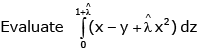along the straight line from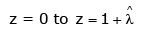Solution:
QUESTION: 5

Consider the differential Equation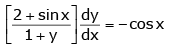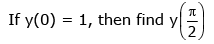Solution:
QUESTION: 6

It is given that  y'' + 2y' + y = 0 y(0) = 0 , y(1) = 0 y(0.5) is _______

Solution:
QUESTION: 7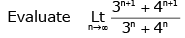Solution:
QUESTION: 8

If the function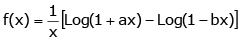is continuous at x = 0 then find f(0)?

Solution:
QUESTION: 9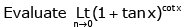Solution:
QUESTION: 10

For what value of k is the following function continuous  x = 0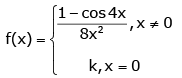Solution:
QUESTION: 11

If y = x |x|, then find Derivative of y?

Solution:
QUESTION: 12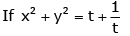and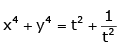find dy/dx

Solution:
QUESTION: 13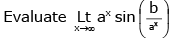Solution:
QUESTION: 14

An insurance company insured 2000 cyclists, 4000 scooter drivers and 6000 motorbike drivers. The probability of an accident involving a cyclist, scooter driver and a motorbike driver are 0.01, 0.03 and 0.15 respectively. One of the insured persons meets with an accident. What is the probability that he is a scooter driver?

Solution:
QUESTION: 15

In answering a question on a MCQ test with 4 choices per question, a student knows the answer, guesses or copies the answer. Let ½ be the probability that he knows the answer, ¼ be the probability that he guesses and ¼ that he copies it. Assuming that a student, who copies the answer, will be correct with the probability ¾, what is the probability that the student knows the answer, given that he answered it correctly?

Solution:
QUESTION: 16

The condition on “a” for which the system of equations

3x − y + 4z = 3, x + 2y − 3z = −2,6x + 5y + az = −3

has a unique solution is

Solution:
QUESTION: 17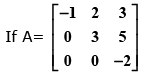then the eigen values of  A3 + 5A + 8I
are

Solution:
QUESTION: 18

The solution of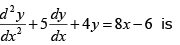Solution:
QUESTION: 19

The differential equation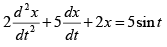given
that x = 2 and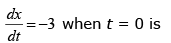Solution:
QUESTION: 20

The next iterative value of the root of x2 − 4 = 0 using the Newton-Raphson method, if the initial guess is 3, is _________

Solution: